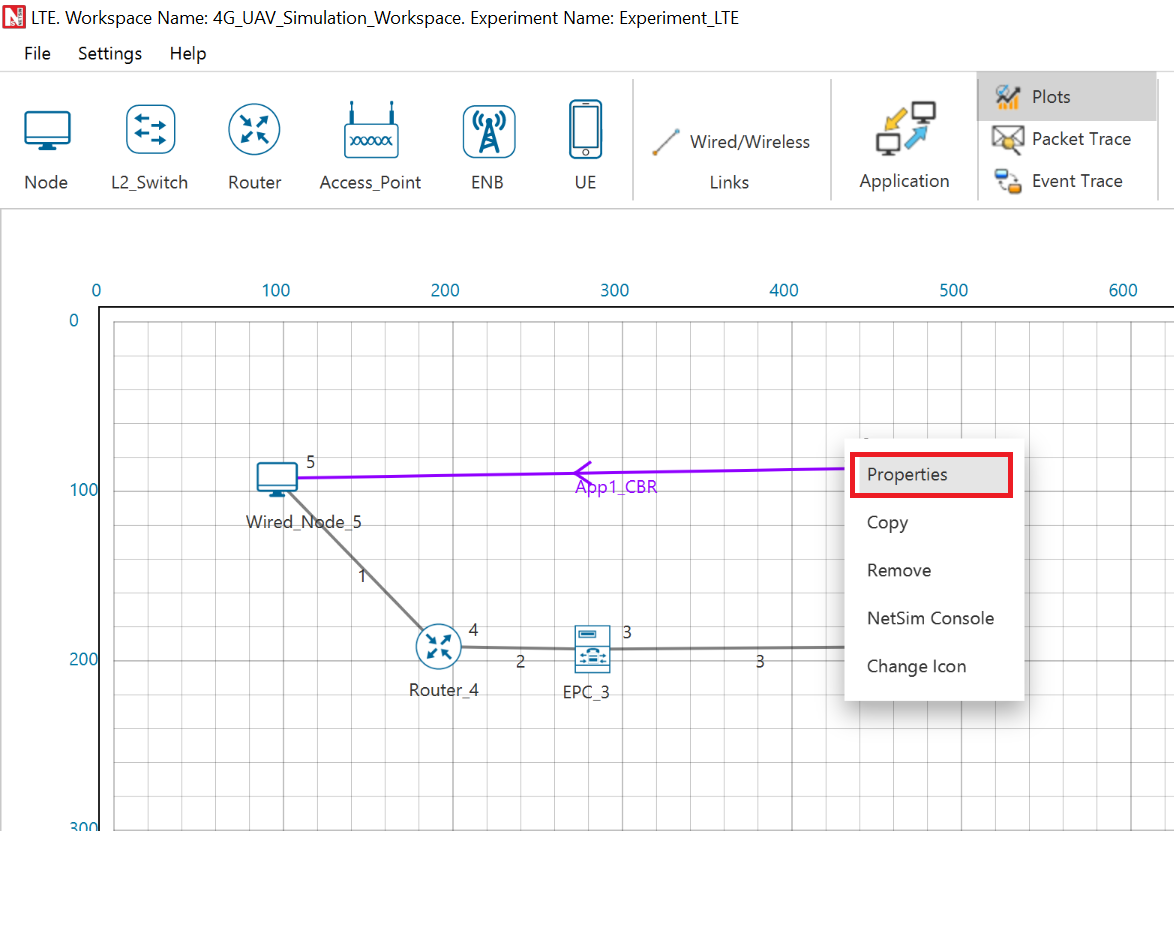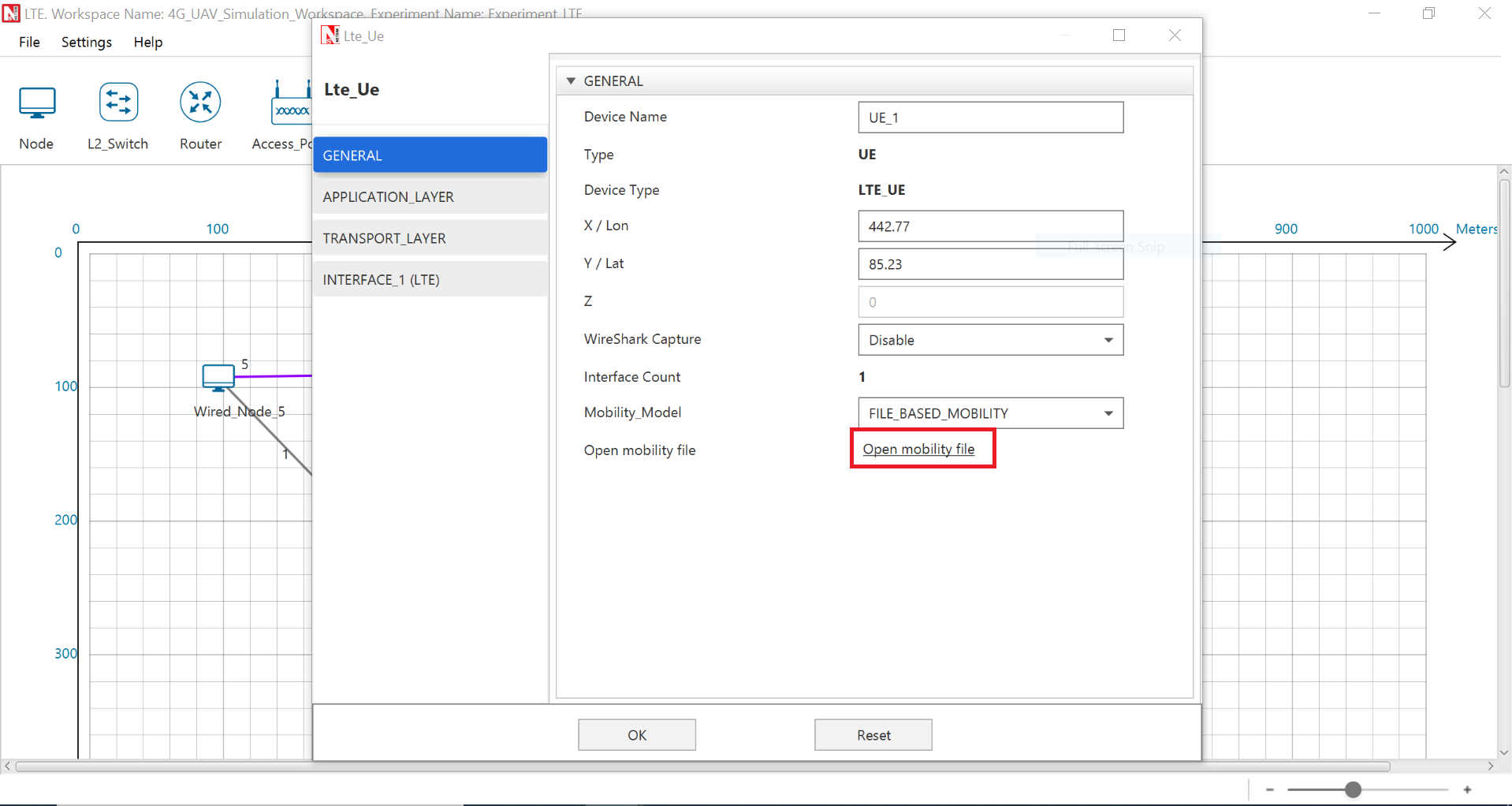To introduce UAV mobility in NetSim, in addition to performing NetSim MATLAB co-simulation,  MATLAB/Simulink UAV models can be simulated separately to obtain the trajectory which can be suitably converted and used as input for NetSim. This can help in accelerating simulations if visualizing the UAV movement is not the primary focus.

To perform this you will have to run UAV Simulation in MATLAB separately before running NetSim Simulation. Here are the steps that are involved in this method.

• Open MATLAB and open the UAV Model. A sample Simulink UAV model file is attached herewith.
• Choose Rapid Accelerator > Click on Run• Once MATLAB Simulation completed, go to MATLAB Desktop and check for results in the Workspace for variables such as tout, North, East, and Height.• The next step is to generate a File-Based Mobility file for NetSim as per its syntax.
```SYNTAX:\$time <time in second> "\$node_(<NetSim Node ID-1>) <X-cordinate> <Y-cordinate> <Z-cordinate>"

Example: For a node in NetSim with Device Id 1, mobility input can be specified as shown below:
\$time 0 "\$node_(0) 120.28 501.15 11.52"
\$time 0.5 "\$node_(0) 135.28 507.73 11.52"
\$time 1.0 "\$node_(0) 133.28 498.15 11.52"```

Based on the MATLAB Simulation data the following code will generate a Mobility.txt (text file) as per the NetSim's mobility file format.

```Code:
fileID=fopen('mobility.txt','w');
for i=1:10001
fprintf(fileID,'\$time %.2f \"\$node_(0) %.2f %.2f %.2f\"\n',tout(i),North(i),East(i),Height(i));
end
fclose(fileID);```

The above code will generate a mobility input for a device with ID 1 in the network Scenario modeled in NetSim. Depending on the Device ID of the node in NetSim for which mobility input is to be generated, the above code can be modified suitably.

For Eg: To generate mobility input for a Node with ID 9, line 4 of the above code can be modified as shown below:

fprintf(fileID,'\$time %.2f \"node_(8) %.2f %.2f %.2f\"\n',tout(i),North(i),East(i),Height(i));The generated mobility file can be given as input to NetSim by following steps.

• NetSim Home Screen > Open the Scenario > Go to UAV Properties• Change Mobility Model from UAV Mobility to File-Based Mobility.• Click on Open Mobility File, copy the content of the mobility file that is generated from MATLAB to this text file.• Run the simulation.
• After Simulation the device mobility can be visualized in NetSim Packet Animator. Note that NetSim animator supports only 2D and hence any movement along the third dimension cannot be visualized. However, the impact of variation in the Z coordinate is factored in the calculations.